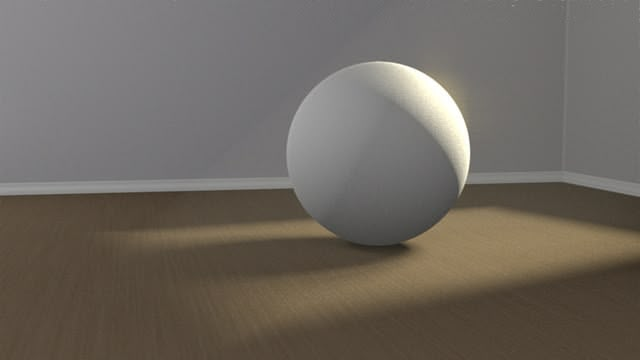(Image by crdotx)

The Xinhua N ewsAgency website is reporting that two Chinese mathematicians, Zhu Xiping and Cao Huaidong, have proved the Poincaré Conjecture. Or rather, I should say, that the proof is currently being scrutinized by a panel from the Asian Journal of Mathematics, where the proposed proof will be published upon consensus.

According to its MathWorld entry, the Poincaré Conjecture states “that the three-sphere is the only type of bounded three-dimensional space possible that contains no holes”, where “a three-sphere is simply a generalization of the usual sphere to one dimension higher”.

The Clay Mathematics Institute also had listed the conjecture as one of its Millenium Problems, each of which carried a one million dollar reward for a proof. Their explanation of the problem puts it this way:

If we stretch a rubber band around the surface of an apple, then we can shrink it down to a point by moving it slowly, without tearing it and without allowing it to leave the surface. On the other hand, if we imagine that the same rubber band has somehow been stretched in the appropriate direction around a doughnut, then there is no way of shrinking it to a point without breaking either the rubber band or the doughnut. We say the surface of the apple is “simply connected,” but that the surface of the doughnut is not. Poincaré, almost a hundred years ago, knew that a two dimensional sphere is essentially characterized by this property of simple connectivity, and asked the corresponding question for the three dimensional sphere (the set of points in four dimensional space at unit distance from the origin). This question turned out to be extraordinarily difficult, and mathematicians have been struggling with it ever since.

As the article makes clear, the movement toward this proof spanned both decades and nationality. American, Russian, and Chinese mathematicians were instrumental in progression of ideas which finally cracked the conjecture. I hope that this example illustrates the global and community effort of current mathematicians in their work.

Congratulations to Zhu Xiping and Cao Huaidong!